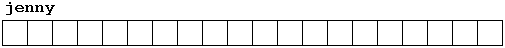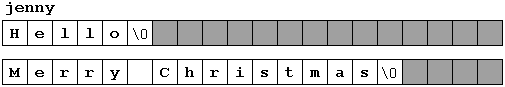# C++ 字符序列

char jenny ;## 初始化以空字符结束的字符序列(Initialization of null-terminated character sequences)

char mystring[] = { 'H', 'e', 'l', 'l', 'o', '\0' };

"the result is: "

char mystring [ ] = { 'H', 'e', 'l', 'l', 'o', '\0' };
char mystring [ ] = "Hello";

mystring = "Hello";
mystring[ ] = "Hello";
mystring = { 'H', 'e', 'l', 'l', 'o', '\0' };

## 给字符序列的赋值

mystring = 'H';
mystring = 'e';
mystring = 'l';
mystring = 'l';
mystring = 'o';
mystring = '\0';

strcpy (string1, string2);

strcpy (mystring, "Hello");

// setting value to string
#include <iostream.h>
#include <string.h>

int main () {
char szMyName ;
strcpy (szMyName,"J. Soulie");
cout << szMyName;
return 0;
}

J. Soulie

// setting value to string
#include <iostream.h>

void setstring (char szOut [ ], char szIn [ ]) {
int n=0;
do {
szOut[n] = szIn[n];
} while (szIn[n++] != '\0');
}

int main () {
char szMyName ;
setstring (szMyName,"J. Soulie");
cout << szMyName;
return 0;
}

J. Soulie

cin.getline ( char buffer[], int length, char delimiter = ' \n');

// cin with strings
#include <iostream.h>

int main () {br />char mybuffer ;
cout << "What's your name? ";
cin.getline (mybuffer,100);
cout << "Hello " << mybuffer << ".\n";
cout << "Which is your favourite team? ";
cin.getline (mybuffer,100);
cout << "I like " << mybuffer << " too.\n";
return 0;
}

Hello Juan.
Which is your favourite team? Inter Milan
I like Inter Milan too.

cin >> mybuffer;

• 它只能接收单独的词(而不能是完整的句子)，因为这种方法以任何空白符为分隔符，包括空格spaces，跳跃符tabulators，换行符newlines和回车符arriage returns。
• 它不能给buffer指定容量，这使得程序不稳定，如果用户输入超出数组长度，输入信息会被丢失。

### 字符串和其它数据类型的转换(Converting strings to other types)

• atoi: 将字符串string 转换为整型int
• atol: 将字符串string 转换为长整型long
• atof: 将字符串string 转换为浮点型float

// cin and ato* functions
#include <iostream.h>
#include <stdlib.h>

int main () {
char mybuffer ;
float price;
int quantity;
cout << "Enter price: ";
cin.getline (mybuffer,100);
price = atof (mybuffer);
cout << "Enter quantity: ";
cin.getline (mybuffer,100);
quantity = atoi (mybuffer);
cout << "Total price: " << price*quantity;
return 0;
}

Enter price: 2.75
Enter quantity: 21
Total price: 57.75

### 字符串操作函数(Functions to manipulate strings)

• strcat: char* strcat (char* dest, const char* src); //将字符串src 附加到字符串dest 的末尾，返回dest。
• strcmp: int strcmp (const char* string1, const char* string2); //比较两个字符串string1 和string2。如果两个字符串相等，返回0。
• strcpy: char* strcpy (char* dest, const char* src); //将字符串src 的内容拷贝给dest，返回dest 。
• strlen: size_t strlen (const char* string); //返回字符串的长度。